# Resources tagged with: Geometric sequences

Filter by: Content type:
Age range:
Challenge level:

There are 28 NRICH Mathematical resources connected to Geometric sequences, you may find related items under Patterns, Sequences and Structure.

Broad Topics > Patterns, Sequences and Structure > Geometric sequences### Tower of Hanoi

##### Age 11 to 14Challenge Level

The Tower of Hanoi is an ancient mathematical challenge. Working on the building blocks may help you to explain the patterns you notice.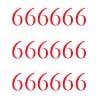### Clickety Click and All the Sixes

##### Age 16 to 18Challenge Level

What is the sum of: 6 + 66 + 666 + 6666 ............+ 666666666...6 where there are n sixes in the last term?### Sixty-seven Squared

##### Age 16 to 18Challenge Level

Evaluate these powers of 67. What do you notice? Can you convince someone what the answer would be to (a million sixes followed by a 7) squared?### Tiny Nines

##### Age 14 to 16Challenge Level

What do you notice about these families of recurring decimals?##### Age 16 to 18Challenge Level

A circle is inscribed in an equilateral triangle. Smaller circles touch it and the sides of the triangle, the process continuing indefinitely. What is the sum of the areas of all the circles?### Converging Product

##### Age 16 to 18Challenge Level

In the limit you get the sum of an infinite geometric series. What about an infinite product (1+x)(1+x^2)(1+x^4)... ?### The Amazing Splitting Plant

##### Age 5 to 7Challenge Level

Can you work out how many flowers there will be on the Amazing Splitting Plant after it has been growing for six weeks?### Vanishing Point

##### Age 14 to 18Challenge Level

How can visual patterns be used to prove sums of series?### Pocket Money

##### Age 11 to 14Challenge Level

Which of these pocket money systems would you rather have?### Double Trouble

##### Age 14 to 16Challenge Level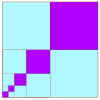### Summing Geometric Progressions

##### Age 14 to 18Challenge Level

Watch the video to see how to sum the sequence. Can you adapt the method to sum other sequences?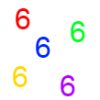### Clickety Click

##### Age 16 to 18 ShortChallenge Level

What is the sum of: 6 + 66 + 666 + 6666 ............+ 666666666...6 where there are n sixes in the last term?### Geometric Parabola

##### Age 14 to 16Challenge Level

Explore what happens when you draw graphs of quadratic equations with coefficients based on a geometric sequence.### Mobile Numbers

##### Age 5 to 11Challenge Level

In this investigation, you are challenged to make mobile phone numbers which are easy to remember. What happens if you make a sequence adding 2 each time?### Production Equation

##### Age 16 to 18Challenge Level

Each week a company produces X units and sells p per cent of its stock. How should the company plan its warehouse space?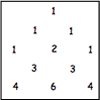### Investigating Pascal's Triangle

##### Age 7 to 11Challenge Level

In this investigation, we look at Pascal's Triangle in a slightly different way - rotated and with the top line of ones taken off.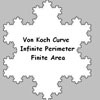### Von Koch Curve

##### Age 16 to 18Challenge Level

Make a poster using equilateral triangles with sides 27, 9, 3 and 1 units assembled as stage 3 of the Von Koch fractal. Investigate areas & lengths when you repeat a process infinitely often.### Sierpinski Triangle

##### Age 16 to 18Challenge Level

What is the total area of the triangles remaining in the nth stage of constructing a Sierpinski Triangle? Work out the dimension of this fractal.### Golden Fibs

##### Age 16 to 18Challenge Level

When is a Fibonacci sequence also a geometric sequence? When the ratio of successive terms is the golden ratio!### Generally Geometric

##### Age 16 to 18Challenge Level

Generalise the sum of a GP by using derivatives to make the coefficients into powers of the natural numbers.### Smaller and Smaller

##### Age 7 to 14Challenge Level

Can you predict, without drawing, what the perimeter of the next shape in this pattern will be if we continue drawing them in the same way?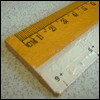### Ruler

##### Age 16 to 18Challenge Level

The interval 0 - 1 is marked into halves, quarters, eighths ... etc. Vertical lines are drawn at these points, heights depending on positions. What happens as this process goes on indefinitely?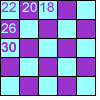### Transformations Tables

##### Age 7 to 11Challenge Level

These grids are filled according to some rules - can you complete them?### Proof Sorter - Geometric Sequence

##### Age 16 to 18Challenge Level

Can you correctly order the steps in the proof of the formula for the sum of the first n terms in a geometric sequence?### Sum the Series

##### Age 16 to 18

This article by Alex Goodwin, age 18 of Madras College, St Andrews describes how to find the sum of 1 + 22 + 333 + 4444 + ... to n terms.### Squaring the Circle and Circling the Square

##### Age 14 to 16Challenge Level

If you continue the pattern, can you predict what each of the following areas will be? Try to explain your prediction.### Binary Squares

##### Age 16 to 18Challenge Level

If a number N is expressed in binary by using only 'ones,' what can you say about its square (in binary)?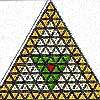### The Great Tiling Count

##### Age 7 to 11Challenge Level

Compare the numbers of particular tiles in one or all of these three designs, inspired by the floor tiles of a church in Cambridge.